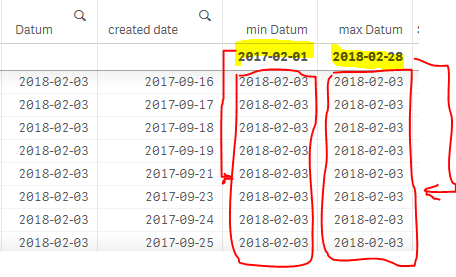# Qlik Sense App Development

Announcements
Make your voice heard! Participate in the 2020 Wisdom of Crowds® Survey. BEGIN SURVEY
New Contributor III

## Min, Max(Datum) in expression, without variables

I have in my scripts an expression with variables, which gives wrong results and I want to rewrite it without variables, but I don't know how to write the MIN and Max of Datum, to obtain the results I need.

In the following example I'll explain what I need from MIN and MAX of Datum. As can be seen in the image bellow, the "min Datum" and "max Datum" are calculated for each row, which is NOT what I need. What I need is to have on each row the MIN and MAX of the selected Datum range. So in this case, the dates highlighted in yellow.

Question 1: How do I write that?Question 2: How do I write the new expression, based on the answer to question 1?

Here are the variables and the expression (to be replaced):

Variables to replace:

 LET vDateStart = '=Num(Min({'& chr(36)& '<%flagDisplay={1}>}Datum))'; LET vDateEnd = '=Num(Max({'& chr(36)& '<%flagDisplay={1}>}Datum))';

Variable to keep:

 SET setActuals = '{\$<%flagDisplay={1}>}';

Expression to rewrite without the variables vDateStart and vDateEnd:

 LET mNewCCount = 'SUM(' & Chr(36) & '(setActuals) IF([created_date] >= ' & Chr(36) & '(vDateStart) AND [created_date] <= ' & Chr(36) & '(vDateEnd), c_distinct_count))';

And here is the NEW expression, without variables (but I don't know how to write the MIN and Max of Datum, to obtain the results I need):

`=SUM(\$(setActuals) IF(num([created_date]) >= num(Min(Datum)) AND num([created_date]) <= num(Max(Datum)), c_distinct_count))`

Tags (2)